# HSSlive: Plus One & Plus Two Notes & Solutions for Kerala State Board

## AP Board Class 7 Maths Chapter 10 Construction of Triangles InText Questions Textbook Solutions PDF: Download Andhra Pradesh Board STD 7th Maths Chapter 10 Construction of Triangles InText Questions Book AnswersAP Board Class 7 Maths Chapter 10 Construction of Triangles InText Questions Textbook Solutions PDF: Download Andhra Pradesh Board STD 7th Maths Chapter 10 Construction of Triangles InText Questions Book Answers

## Andhra Pradesh State Board Class 7th Maths Chapter 10 Construction of Triangles InText Questions Books Solutions

 Board AP Board Materials Textbook Solutions/Guide Format DOC/PDF Class 7th Subject Maths Chapters Maths Chapter 10 Construction of Triangles InText Questions Provider Hsslive

2. Click on the Andhra Pradesh Board Class 7th Maths Chapter 10 Construction of Triangles InText Questions Answers.
3. Look for your Andhra Pradesh Board STD 7th Maths Chapter 10 Construction of Triangles InText Questions Textbooks PDF.
4. Now download or read the Andhra Pradesh Board Class 7th Maths Chapter 10 Construction of Triangles InText Questions Textbook Solutions for PDF Free.

## AP Board Class 7th Maths Chapter 10 Construction of Triangles InText Questions Textbooks Solutions with Answer PDF Download

Find below the list of all AP Board Class 7th Maths Chapter 10 Construction of Triangles InText Questions Textbook Solutions for PDF’s for you to download and prepare for the upcoming exams:

Look at the picture and answer the following questions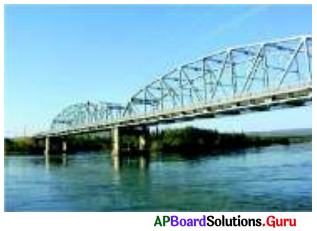Question 1.
Name some things from your daily life that look like triangles.
Samosa, chapathi, window elevations, house tops, bridge trusses, floor tessellations.

Question 2.
What are the types of triangles can see in the picture?
Right triangles,
Equilateral triangles.

Question 3.
Do you think all triangles shown are similar with their properties? What are they?
Yes. All right triangles are similar.

All triangles are similar because they have
(a) right angle
(b) equal side and
(c) equal hypotenuse.

Check Your Progress [Page No. 69]

Question 1.
Construct an equilateral triangle ∆XYZ with XY = 4 cm.
Given sides of an equilateral triangle ∆XYZ is XY = 4 cm.
In equilateral triangle all sides ,gresequal in length
∴ XY = YZ = ZX = 4 cm.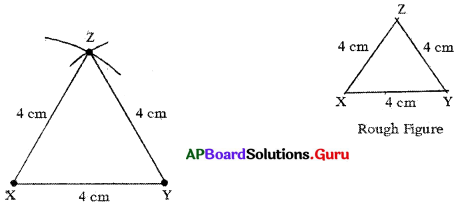Steps of Construction:

1. Draw a rough sketch of the triangle and label it with the given measurements.
2. Draw a line segment with XY = 4 cm,
3. Draw an arc with centre X and radius 4 cm.
4. Draw another arc with centre Y and radius 4 cm to intersecting the previous arc at Z.
5. Join XZ andYZ.

Hence, required ∆XYZ is constructed with the given measurements.

Question 2.
Construct an isosceles triangle ∆PQR With PQ = PR = 3 cm, QR = 5 cm.
Given sides of an isosceles triangle ∆PQR are PQ = PR = 3 cm and QR = 5 cm
In isosceles triangle two sides are equal.
∴ PQ = PR = 3 cm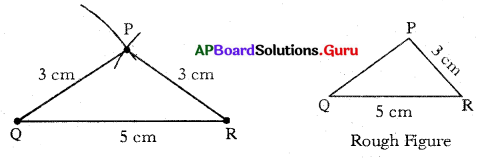Steps of construction:

1. Draw a rough sketch of the triangle and label it with the given measurements.
2. Draw a line segment QR = 5 cm.
3. Draw an arc with centre Q and radius 3 cm.
4. Draw another arc with centre R and same radius (3 cm) to intersect the previous arc at P.
5. Join PQ and PR.

Hence, required APQR is constructed with the given measurements.

Let’s Think [Page No. 69]

Can you construct ∆ABC with AB = 4 cm, BC = 5 cm and CA =10 cm? Why? Justify your answer.
Given sides of a ∆ABC are AB = 4 cm, BC = 5 cm and CA =10 cm.
In any triangle sum of any two sides is always greater than the third side.
AB + BC = 4 cm + 5 cm = 9 cm <10 cm.
Sum of AB + BC < AC
So, with the given measurements construction of ∆ABC is not possible.

Check Your Progress [Page No. 72]

Question 1.
Construct ∆MAT with measurements MA = 5.5 cm, MT = 4 cm and ∠M=70°.
Given measurements of ∆MAT are MA = 5.5 cm, MT = 4 cm and ∠M = 70°.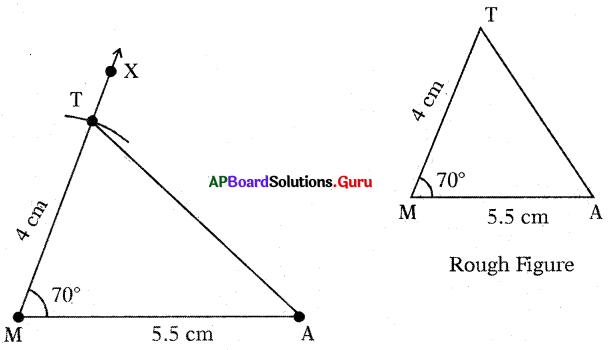Steps of Construction:

1. Draw a rough sketch of the triangle and label it with the given measurements.
2. Draw a line segment with MA = 5.5 cm.
3. Draw a ray MX−→− such that ∠AMX = 70°
4. Draw an arc with centre M and radius 4 cm, to intersect the MX−→− at point T.
5. Join AT.

Hence, required ∆MAT is constructed with the given measurements.

Let’s Explore [Page No. 72]

Question 1.
Construct the triangle with the measurements of AB = 7cm, ∠B = 60° and ∠C = 70°.
Given measurements of ∆ABC are AB = 7 cm, ∠B = 60° and ∠C = 70°.
In ∆ABC, ∠A + ∠B + ∠C = 180°
∠A + 60° + 70° = 180° .
∠A + 130° = 180°
∠A = 180° – 130° = 50°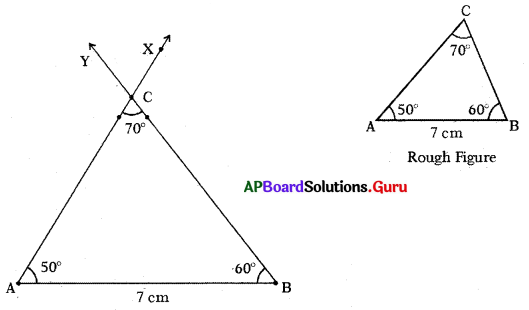Steps of Construction:

1. Draw a rough sketch of triangle and label it with given measurements.
2. Draw a line segment with AB = 7 cm.
3. Draw a ray BY such that ABY = 60°.
4. Draw another ray AX such that BAX = 50°.
5. Name the intersecting of rays AX and BY is C.

Hence, required ∆ABC is constructed with the given measurements.

Check Your Progress [Page No. 73]

Question 1.
Construct ∆ABC with the measurements ∠A = 90°, ∠C = 50° and AC = 8 cm.
Given measurements of ∆ABC are ∠A = 90°, ∠C = 50° and AC = 8 cm.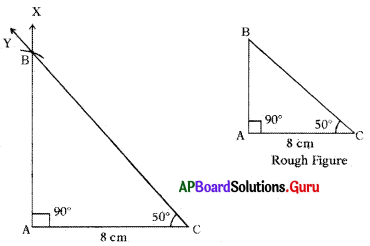Steps of Construction:

1. Draw a rough sketch of triangle and label it with given measurements.
2. Draw a line segment with AC = 8 cm.
3. Draw a ray AX such that ∠CAX = 90°.
4. Draw another ray CY such that ∠ACY = 50°.
5. Name the intersecting point of AX and CY as B.

Thus, required ∆ABC is constructed with the given measurements.

Let’s Think [Page No. 73]

Construct a triangle with angles 100°, 95° and a side of length of your choice. Can1 you construct the triangle?
No. It is not possible. We know that the sum of the angles of a triangle is 180°.
But, given two angles are 100° and 95°. Their sum is greater than 180°.
So, we cannot construct the triangle.

Examples:

Question 1.
Construct ∆ABC with slides AB = 6 cm, BC = 4 cm and AC = 5 cm.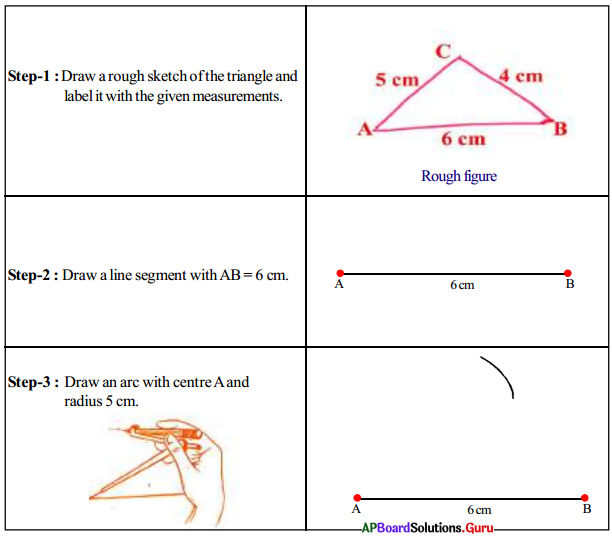Question 2.
Construct ∆EFD with the measurements ∠F = 70°, EF = 4 cm and FD = 5 cm.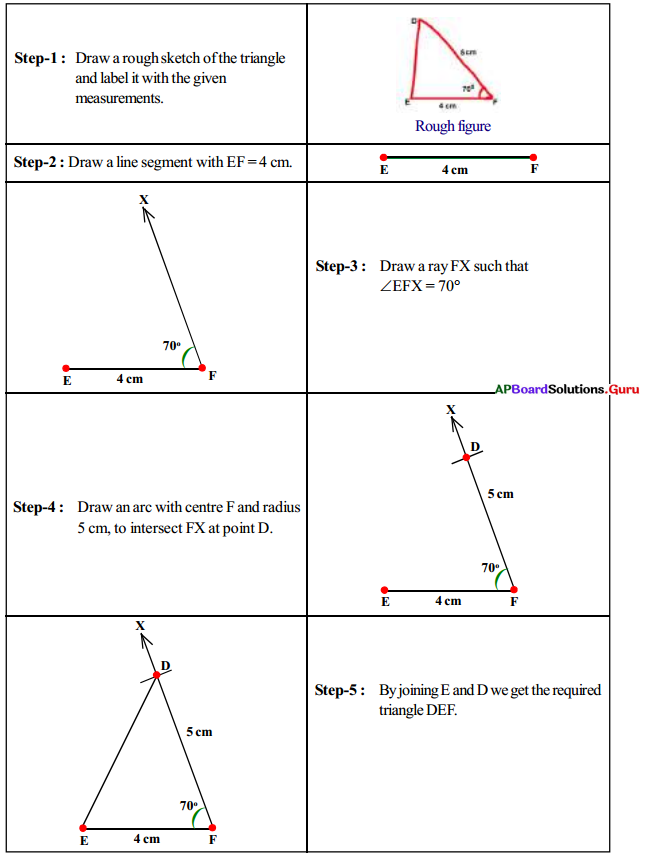Question 3.
Construct ∆ABC with AB = 6 cm, BC = 7 cm, ∠BAC = 80°.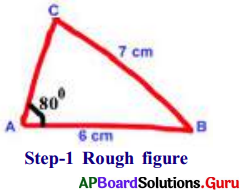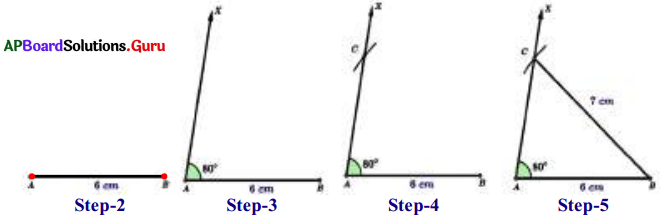• Step-1: Draw a rough sketch of ∆ABC and label it with the given measurements.
• Step-2: Draw a line segment AB of length 6 cm.
• Step-3: Draw a ray AX such that ∠BAX = 80°.
• Step-4: Draw an arc with center B and radius 7 cm to Intersect the ray AX at C.
• Step-5: Join B and C to get the required ∆ABC.

Question 4.
Construct a right angled triangle ∆XYZ, XY = 4 cm, XZ = 6.5 cm and ∠Y = 90°.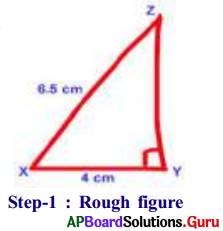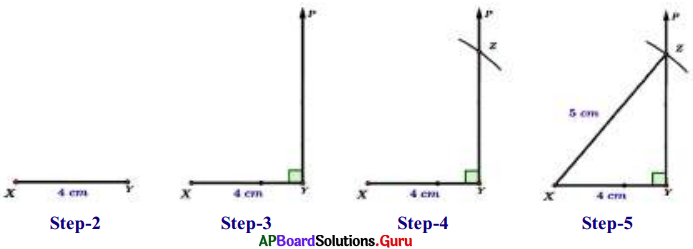• Step-1: Draw a rough sketch of ∆XYZ and label it with given measurements.
• Step-2: Draw a line segment with XY = 4 cm.
• Step-3: Draw a ray YP such that ∠XYP = 90°.
• Step-4: Draw an arc with center X and radius 6.5 cm to intersect the Ray YP at Z.
• Step-5: Join X and Z to get the required ∆XYZ.

Question 5.
Construct ∆PEN with measurements PE. = 7 cm, ∠PEN = 25° and ∠EPN = 60°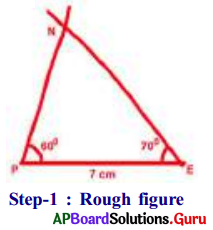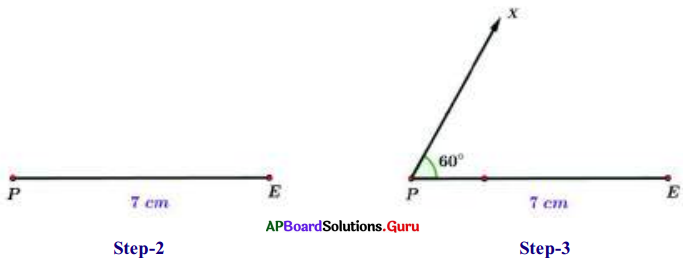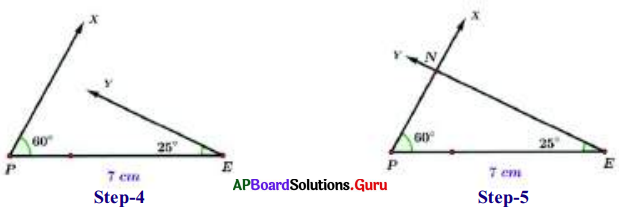• Step-1: Draw a rough sketch of the triangle and label it with given measurements.
• Step-2: Draw a line segment with PE = 7 cm,
• Step-3: Draw a ray PX such that ∠EPX = 60°.
• Step-4: Draw another ray EY such that ∠PEY = 25°.
• Step-5: Rays PX and EY intersect each other at N.

Hence, we get the required ∆PEN.
(If necessary, extend the rays to form a triangle.)

Question 6.
Construct ∆MPC the triangle with the measurements MP = 4 cm, ∠P = 45° arid ∠C = 80°.
Now we have to find out the third angle.
We know that the sum of three angles in a triangle is 1800.
So, ∠M + ∠P + ∠C = 180°
⇒ ∠M + 450 + 80° = 180°
⇒ ∠M + 125° = 180°
⇒ ∠M = 180 – 125 = 55°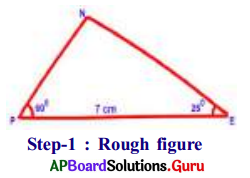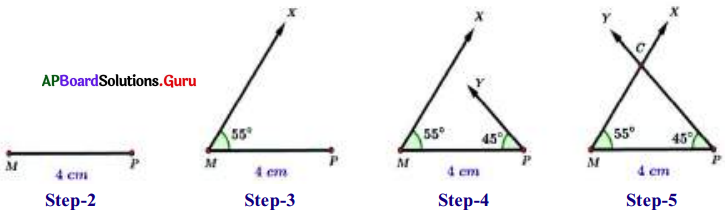• Step-1: Draw a rough sketch of the triangle and label it with given measurements.
• Step-2: Draw a line segment with MP = 4 cm.
• Step-3: Draw a ray MX such that ∠PMX = 55°.
• Step-4: Draw another ray PY such that ∠MPY = 45°.
• Step-5: Rays MX and PY intersect each other at C. (If necessary extend the rays to ‘ form a triangle.)

Hence, we get the required ∆MPC.
Verification: By using the protractor and check whether ∠C is equal to 80° or not.

Practice Questions [Page No. 78]

Find the number of triangles in the given figures.

Question 1.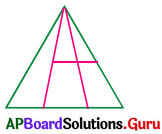(a) 8
(b) 9
(c) 10
(d) 12
(b) 9

Explanation: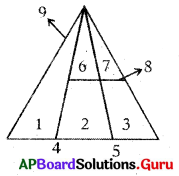Question 2.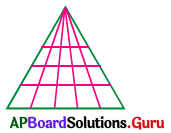(a) 60
(b) 65
(c) 84
(d) 90
(a) 60

Explanation: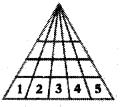Number of rows = 4
Sum of numbers in each row = 1 + 2 + 3 + 4 + 5 = 15
Number of triangles in the picture = 4 × 15 = 60

Question 3.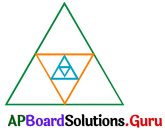(a) 12
(b) 13
(c) 14
(d) 15
(b) 13

Explanation:
Formula to count number of triangles = 4n + 1
Here n = number of embedded triangles in outer triangle
In the figure n = 3
So, number of triangles = 4(3) + 1 = 12 + 1 = 13

Question 4.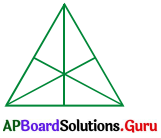(a) 16
(b) 13
(c) 9
(d) 7
(a) 16

Question 5.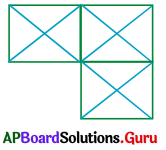(a) 21
(b) 23
(c) 25
(d) 29
(d) 29

Explanation: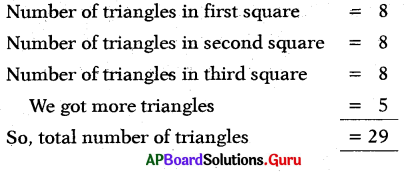Question 6.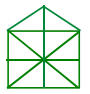(a) 10
(b) 19
(c) 21
(d) 23
(c) 21

Explanation: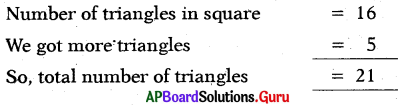Question 7.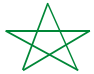(a) 5
(b) 6
(c) 8
(d) 10
(c) 8

Question 8.(a) 9
(b) 10
(c) 11
(d) 12
(a) 9

Explanation: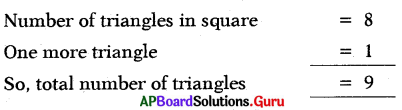Question 9.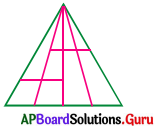(a) 19
(b) 20
(c) 16
(d) 14
(a) 19

Question 10.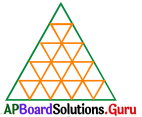(a) 56
(b) 48
(c) 32
(d) 60
(b) 48

Explanation:
Formula to count number of triangles = n(n+2)(2n+1)8
Where n = number of thangles formed in a side
Here in the given figure n = 5
Number of triangles = 5(5+2)(2×5+1)8=5×7×118 = 48.1
So, total number of triangles = 48.

## Andhra Pradesh Board Class 7th Maths Chapter 10 Construction of Triangles InText Questions Textbooks for Exam Preparations

Andhra Pradesh Board Class 7th Maths Chapter 10 Construction of Triangles InText Questions Textbook Solutions can be of great help in your Andhra Pradesh Board Class 7th Maths Chapter 10 Construction of Triangles InText Questions exam preparation. The AP Board STD 7th Maths Chapter 10 Construction of Triangles InText Questions Textbooks study material, used with the English medium textbooks, can help you complete the entire Class 7th Maths Chapter 10 Construction of Triangles InText Questions Books State Board syllabus with maximum efficiency.

## FAQs Regarding Andhra Pradesh Board Class 7th Maths Chapter 10 Construction of Triangles InText Questions Textbook Solutions

#### Can we get a Andhra Pradesh State Board Book PDF for all Classes?

Yes you can get Andhra Pradesh Board Text Book PDF for all classes using the links provided in the above article.

## Important Terms

Andhra Pradesh Board Class 7th Maths Chapter 10 Construction of Triangles InText Questions, AP Board Class 7th Maths Chapter 10 Construction of Triangles InText Questions Textbooks, Andhra Pradesh State Board Class 7th Maths Chapter 10 Construction of Triangles InText Questions, Andhra Pradesh State Board Class 7th Maths Chapter 10 Construction of Triangles InText Questions Textbook solutions, AP Board Class 7th Maths Chapter 10 Construction of Triangles InText Questions Textbooks Solutions, Andhra Pradesh Board STD 7th Maths Chapter 10 Construction of Triangles InText Questions, AP Board STD 7th Maths Chapter 10 Construction of Triangles InText Questions Textbooks, Andhra Pradesh State Board STD 7th Maths Chapter 10 Construction of Triangles InText Questions, Andhra Pradesh State Board STD 7th Maths Chapter 10 Construction of Triangles InText Questions Textbook solutions, AP Board STD 7th Maths Chapter 10 Construction of Triangles InText Questions Textbooks Solutions,
Share: